# Lookup to left with VLOOKUP function in Excel

In this article, we will learn how to Lookup to left with the VLOOKUP function in excel.

Scenario:

For Fact, the VLOOKUP function looks to the right of the lookup array. It is one of its limitations. But its not easy to change the table, but we can make the formula work the other way. Learn more about how to perform VLOOKUP function to lookup left here below.

How to Solve the Problem?

For the formula to understand first we need to revise a little about the following functions

Now we will make a formula using the above functions. The CHOOSE function will return the table with required field on the right. The VLOOKUP function gets the argument table array in the right format. Now select the right column num as per required field.

Generic Formula:

= VLOOKUP ( lookup_value , CHOOSE ( { 1 , 2 } , lookup_range , req_range ) , 2 , 0)

lookup_value : value to look for

lookup_range : range, where to look lookup_value

req_range : range, where corresponding value is required

2 : second column, num representing the req_range

0 : look for the exact match

Example:

The above statements can be confusing to understand. So let’s understand this by using the formula in an example

Here we have a table having details about the world's countries, continent and code. We need to find the country name from the given country code. Here the problem is Country column is left of the code column. So we will use the above Generic formula.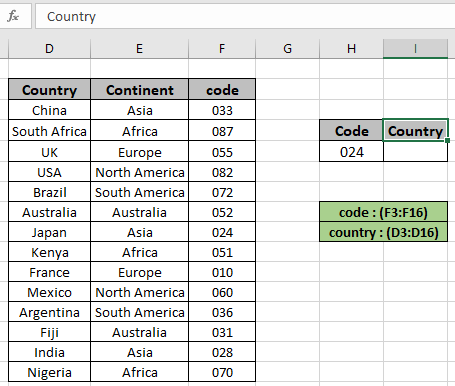Named range used for range (code - F3:F16) & range (country - D3:D16 ) array. Here we used the named range instead for cell reference array as it's easy to understand. FIrst We need to look for a country name where country code is given in H5 cell.
Use the formula in the G6 cell:

= VLOOKUP ( H5 , CHOOSE ( { 1 , 2 }, code , country) , 2 , 0 )

Named ranges

code : (F3:F16)

country : (D3:D16)

Explanation:

• CHOOSE ( { 1 , 2 }, code , country) will form its own new table array as shown below.

{ 33 , "China" ; 87 , "South Africa" ; 55 , "UK" ; 82 , "USA" ; 72, "Brazil" ; 52 , "Australia" ; 24 , "Japan" ; 51 , "Kenya" ; 10 , "France" ; 60 , "Mexico" ; 36 , "Argentina" ; 31 , "Fiji" ; 28 , "India" ; 70 , "Nigeria" }

• VLOOKUP function looks up for the country code in the first column of the given table argument and returns the result from the second column as given.
• 0 used to look for the exact match.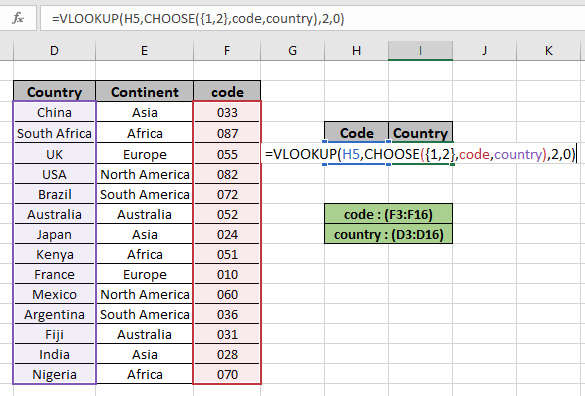The formula looks like as shown in the snapshot above. Click Ok to get the country name corresponding to code 024.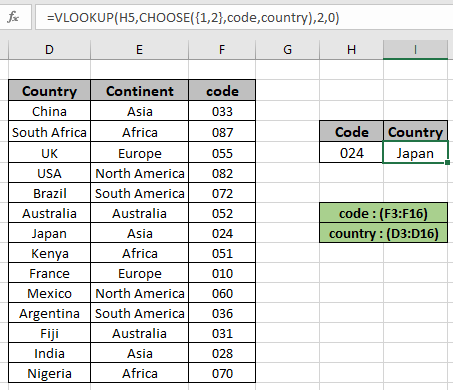As you can see from the above snapshot we obtained the country Japan matching the code value in the formula. Now if you wish to extract Continent name from the code value.
Use the formula:

= VLOOKUP ( H5 , CHOOSE ( { 1 , 2 }, cont , country) , 2 , 0 )

Named ranges

code : (F3:F16)

cont : (E3:E16)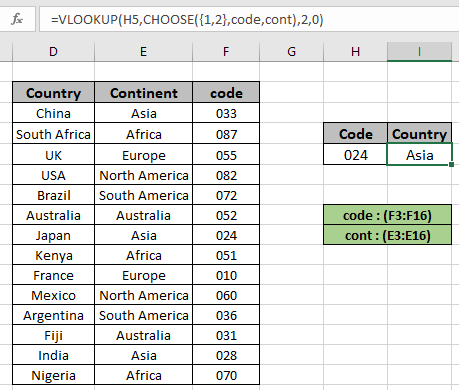You can see from the above snapshot that this formula works fine.
Generally we use the combination of INDEX & MATCH function to get the value from the left of the lookup array.
Use the formula:

=INDEX ( D3:F16 , MATCH ( H6 , code , 0 ) , 2 )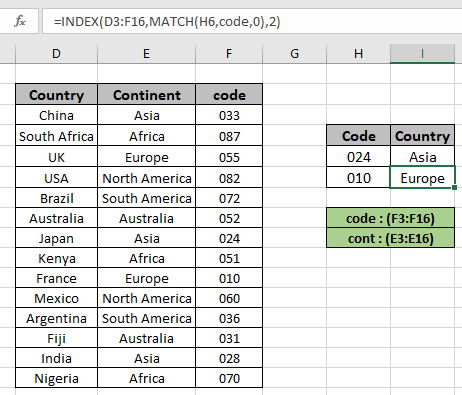As you can see from the above snapshot we obtained all the details matching the code value from the table. Extract details from the table using the above stated formula. Here are some observational noter while using the above formula.

Notes:

1. Value of col_argument cannot be smaller than 1 or greater than number of columns
2. The function returns the #NA error if the lookup array argument to the VLOOKUP function is not of same length of the table array.
3. The formula returns an error if lookup_value doesn't match the value in the table lookup_array.
4. The function matches exact value as the match type argument to the VLOOKUP function is 0.
5. The lookup values can be given as cell reference or directly using quote symbol ( " ) in the formula as arguments.

Hope you understood how to Lookup nth match in table using INDEX & MATCH function. Explore more articles on Excel lookup value here. Please feel free to state your queries below in the comment box. We will certainly help you. If you liked our blogs, share it with your friends on Facebook. And also you can follow us on Twitter and Facebook. We would love to hear from you, do let us know how we can improve, complement or innovate our work and make it better for you. Write us at info@exceltip.com

Related Articles

Use INDEX and MATCH to Lookup Value : INDEX & MATCH function to look up value as required.

SUM range with INDEX in Excel : Use INDEX function to find the SUM of the values as required.

How to use the INDEX function in Excel : Find the INDEX of array using the INDEX function explained with example.

How to use the MATCH function in Excel : Find the MATCH in the array using the INDEX value inside MATCH function explained with example.

How to use LOOKUP function in Excel : Find the lookup value in the array using the LOOKUP function explained with example.

How to use the VLOOKUP function in Excel : Find the lookup value in the array using the VLOOKUP function explained with example.

Popular Articles

50 Excel Shortcut to Increase Your Productivity

Edit a dropdown list

Absolute reference in Excel

If with wildcards

Vlookup by date

Terms and Conditions of use

The applications/code on this site are distributed as is and without warranties or liability. In no event shall the owner of the copyrights, or the authors of the applications/code be liable for any loss of profit, any problems or any damage resulting from the use or evaluation of the applications/code.